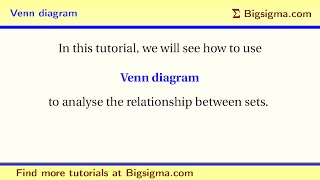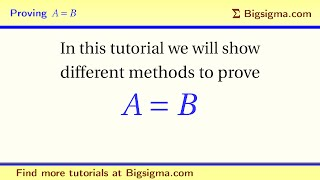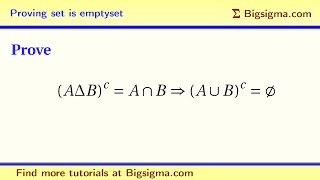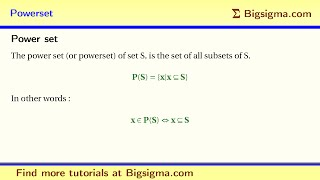Sets and Venn Diagrams

In this demo, we will see how to use Venn diagram to analyze the relationship between sets.Equality of Sets

In this tutorial, we learn how to prove equality of sets.Proving set is emptyset

In this tutorial, we will learn how to prove that a set is empty. We will also learn how to handle complementary set.Power Sets

The power set (or powerset) of set S is a set of all subsets of S. In this tutorial, we will learn to use this definition.